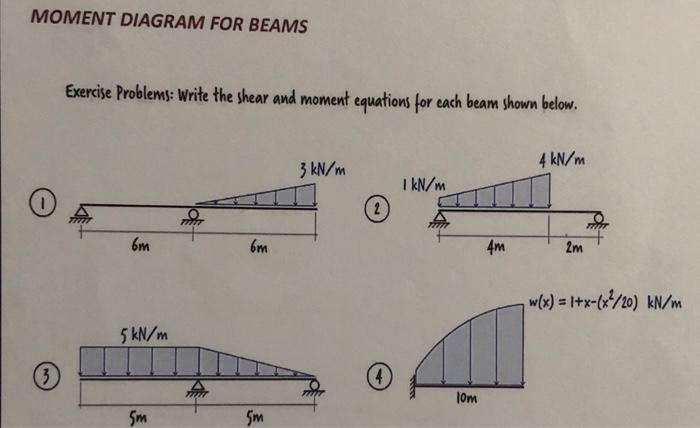# Write the shear and moment equations for each beam shown below

Question-AnswerCategory: Strength of MaterialsWrite the shear and moment equations for each beam shown below

Write the shear and moment equations for each beam shown below